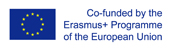This project has been funded with support from the European Commission.
This web site reflects the views only of the author, and the Commission cannot be held responsible for any use which may be made of the information contained therein.

Select language   >   IT EN RO PL LT

### Pythagorean theorem

Subject taught
- Math

Type of Product
- Exercises
- Slides

Language Skills Developed
- Speaking
- Writing

Transferable/Scientific Skills Developed
Student will develop self-motivation, analytical skills, problem-solving skills

Description
General aims: :

Student will be able to explain how Pythagoras theorem works.
Student will be able to solve basic mathematical problems connected with Pythagoras theorem which can be used in the real life. He will know basic vocabulary in English connected with geometry. Student will develop positive learning habits such as organisational and problem-solving skills.

Linguistic aims:
- to read English with ease
- to understanding content
- to learn from written texts
- to master tools for gathering information
- to express understanding and experiences
- to enable students to understand topic correctly
- to translate words connected with percentages in English into the mother tongue.

Subject specific aims:
- student can explain how Pythagoras theorem works
- student is able to use Pythagoras theorem in math problems which are connected directly with a right triangle
- student knows how to apply Pythagoras’ Theorem to 2D problems
- student knows common ‘Pythagorean triples’
- student knows what is meant by given an answer in ‘exact form’
- student knows how to find the perpendicular height and area of an equilateral triangle
- student can solve problems using Pythagoras theorem even if they aren´t directly connected to a right triangle.

Target group age:
Students from primary school 13-14 years old. What is more, these slides can be used by anyone who wants to do extend one’s knowledge about Pythagoras theorem .

Level of competence in English (CEFR)
B1/B1+

Time required to use the resource with the students:
The material can be divided. This way the teacher can use the resource with the students for 3-4 lessons. There are 32 slides.

How to use it
The teacher can use the slides during the lesson to introduce the Pythagoras theorem or to practice it. There are exercises which students can do indyvidually or in pairs. There are methods, exercises in which students can test themselves, am game. The students give the answers with the examples. The teacher should point the good answers on the slides and show students the examples from the resource to visualize the most important issues and definitions. The teacher highlights some new words for students in English and students should find the translation these words into the mother tongue and write down the new words in English in the notebook with the right translation.

The teacher can recommend and encourage the students to get to know more about Pythagorean theorem using the resource at home.
Possible difficulties for the students
Students who have poor knowledge of English can have problems with understanding the content. Students who has problems doing basic operations with powers and roots can face difficulties in solving math problems with the Pythagorean theorem which are introduced on the slides which is recommended.